# 后台站点文件扫描

## 遍历文件

### scandir

#### 给定目录

<?php
/**
* Created by PhpStorm.
* User: ${郭璞} * Date: 2017/2/3 * Time: 20:25 * Description: scandir函数测试 */$pathinfo = scandir('.');
var_dump($pathinfo); 效果如下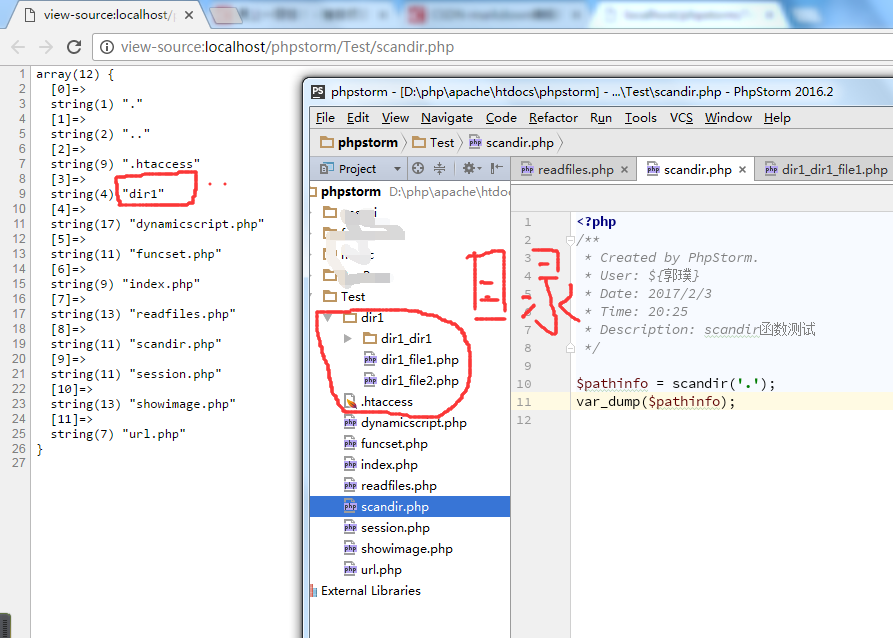#### 非法使用 所谓非法使用，就是指给出非目录文件时的场景，比如我们直接给个文件的路径，就是这样了。 <?php /** * Created by PhpStorm. * User:${郭璞}
* Date: 2017/2/3
* Time: 20:25
* Description: scandir函数测试
*/

$pathinfo = scandir('./scandir.php'); var_dump($pathinfo);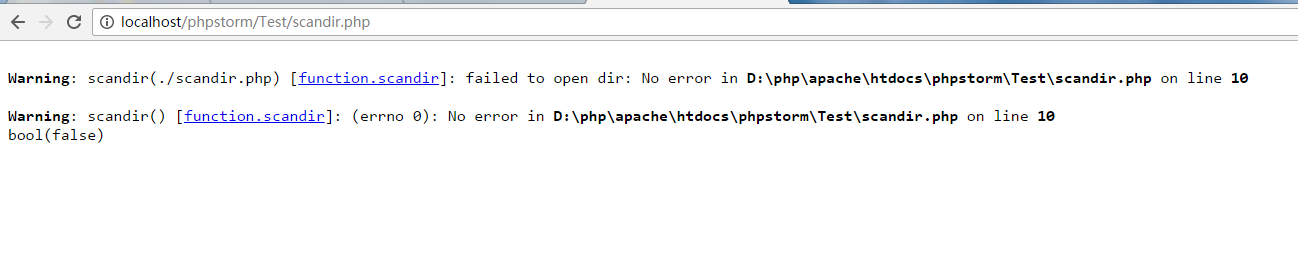### dir函数

<?php
/**
* Created by PhpStorm.
* User: ${郭璞} * Date: 2017/2/3 * Time: 20:25 * Description: scandir函数测试 */$path = ".";
if(is_dir($path)) {$dirinfo = dir($path); while($file = $dirinfo->read()) { echo "<mark>".$file."</mark><br />";
}
$dirinfo->close(); }else if (is_file($path)) {
echo "<font color='green'>".$path."</font>"; }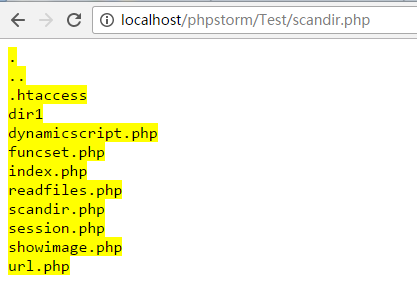如果将$path='.'换成$path='./scandir.php'。将出现如下结果。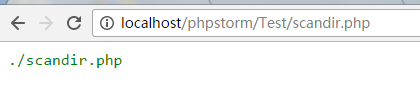当然，这两个方法都没能实现我们想要的效果。不能突破子目录的情况，没办法遍历到最底层的文件信息。 ### 递归法 既然如此，那就得另寻他法了。我个人觉得递归的方法不赖，应该可以灵活地处理这些问题，说做就做。 使用面向过程的PHP编码方法需要处理外部数组引用问题，显得代码不是很容易理解，所以我选择面向对象的方法，将外部数组封装到一个类中，专门用于处理这类问题。 <?php /** * Created by PhpStorm. * User:${郭璞}
* Date: 2017/2/3
* Time: 9:32
* Description: 读取给定目录及子目录下文件路径信息
*/

class FileWatcher{
public $fileinfo; /** * FileWatcher constructor. * @param$path 给定路径
*/
function __construct(){
$this->fileinfo = array(); } /** * 去除路径设置信息，析构方法 */ function __destruct() { // TODO: Implement __destruct() method.$this->fileinfo = null;
}

public function scanDir($path) { if(is_dir($path)) {
$tmpdir = dir($path);
while($tmpfile =$tmpdir->read()) {
if($tmpfile!='.' &&$tmpfile!='..')
$this->scanDir($path."/".$tmpfile); }$tmpdir->close();
}
if(is_file($path)) { array_push($this->fileinfo, $path); } return$this->fileinfo;
}

}

$fileWatcher = new FileWatcher();$result = $fileWatcher->scanDir('.'); var_dump($result);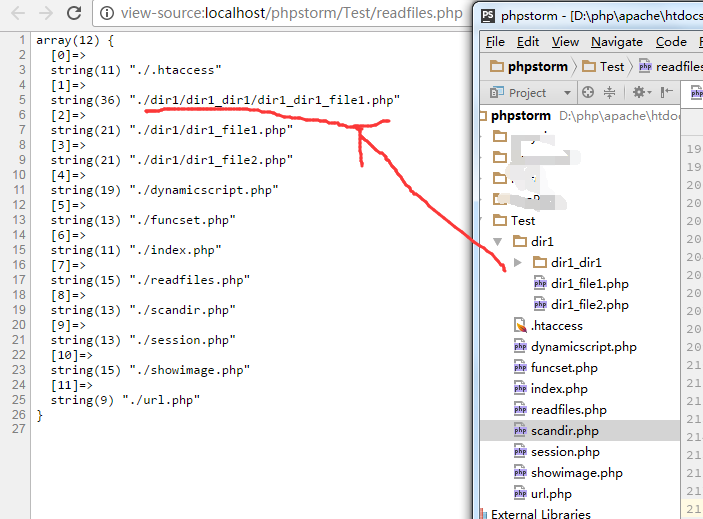## 路径解析

### 数据结构设计

class FileInfo{
// 目录深度
public $level; // 文件经过的路径，以数组形势依次填充 public$pathstep;
// 文件的完整路径
public $fullpath; public function __construct() { //pathstep 存储当前路径经过的文件夹信息$this->pathstep = array();
}

public function __destruct()
{
// TODO: Implement __destruct() method.
$this->pathstep = null;$this->level = null;
$this->fullpath = null; } } ### 原理解析 我个人认为原理还是比较简单的了，那就是以文件分隔符作为计算标准。当然了，需要处理一大堆的路径适配问题，尤其是./../这样的相对路径。 处理完这些之后就轻松多了，使用explode函数将字符串进行分割，装填到数组中即可。 ### 代码实现 class PathParser{ private$patharray;

private $resultSet; public function __construct($patharray)
{
// 从外部获取到处理结果集
$this->patharray =$patharray;
// 初始化结果集数组
$this->resultSet = array(); // bean类对象$this->fileinfo = new FileInfo();
}

public function __destruct()
{
// TODO: Implement __destruct() method.
$this->resultSet = null;$this->level = null;
$this->fullpath = null; } public function parse() { for ($index=0; $index<count($this->patharray); $index++) { // 赋予完整路径$fileinfo = new FileInfo();
$fileinfo->fullpath =$this->patharray[$index]; //计算level$fileinfo->level = $this->parseLavel($fileinfo->fullpath);
//            echo $fileinfo->level."<------->"; // 计算经过的路径并进行存储$fileinfo->pathstep = $this->parseStep($fileinfo->fullpath);
//            echo $fileinfo->pathstep."<br />"; // var_dump($fileinfo->pathstep);

array_push($this->resultSet,$fileinfo);

}
//返回计算结果，整体作为结果集返回
return $this->resultSet; } /** * 获取给定路径所经过的路径的结果集，将用于分级目录展示 * @param$fileinfo
* @return int
*/
public function parseStep($fileinfo) { if(!$fileinfo) {
echo "<mark>".$fileinfo." path error!</mark>"; exit(); } // 判断是否为相对路径是的话去掉第一级目录。 啊好烦，windows上和linux上差别还这么大，怎么处理好呢。。。 // 还是按照文件在服务器上的位置来进行来处理好了。判断是不是相对路径然后再针对“路径分隔符”计算路径的level if($this->isRelativePath($fileinfo) == 1) { // 相对路径处理 // 去掉相对路径符号$fileinfo = substr($fileinfo,2, strlen($fileinfo));

// 按照目录分隔符 作为切割标准，结果就是路径本身包含的路径信息
return explode("/", $fileinfo); }else if($this->isRelativePath($fileinfo) == 2){$fileinfo = substr($fileinfo, 3, strlen($fileinfo));
return explode("/", $fileinfo); }else if ($this->isAbsolutePath($fileinfo)) { // 绝对路径处理 }else{ // 文件路径非法 echo "<mark>".$fileinfo." 文件路径非法</mark>";
exit();
}
}

public function parseLavel($fileinfo) { if(!$fileinfo) {
echo "<mark>".$fileinfo." path error!</mark>"; exit(); } //按照文件在服务器上的位置来进行来处理好了。判断是不是相对路径然后再针对“路径分隔符”计算路径的level if($this->isRelativePath($fileinfo) == 1) { // 相对路径处理 // 去掉当前相对路径符号$fileinfo = substr($fileinfo,2, strlen($fileinfo));
// 通过计算 路径分隔符来作为level的判断标准
//            echo "<mark>".count(explode("/", $fileinfo))."</mark>"; return count(explode("/",$fileinfo));
}else if ($this->isRelativePath($fileinfo) == 2 ) {
//去掉父级目录信息
$fileinfo = substr($fileinfo, 3, strlen($fileinfo)); return count(explode("/",$fileinfo));
}else if ($this->isAbsolutePath($fileinfo)) {
// 绝对路径处理
// 算了，先不做这块了，貌似偏离了我这个需求。
}else{
// 文件路径非法
echo "<mark>".$fileinfo." 文件路径非法</mark>"; exit(); } } /** * 判断是否为相对路径 * @param$path
* @return bool
*
*/
private function isRelativePath($path) { // 父级目录拥有更高的优先级$prefix = substr($path, 0, 3); if($prefix == "../") {
return 2;
}

// 处理 当前目录情况
$prefix = substr($path, 0, 2);
if ($prefix == "./"){ return 1; }else{ return false; } } /** * 判断给定路径是否为绝对路径 * @param$path
* @return bool
*/
private function isAbsolutePath($path) {$prefix = substr($path, 0, 1); if($prefix=="/"){
return true;
}else{
return false;
}
}

}

## 演示

### 当前目录


//获取全部文件以及路径信息
$fileWatcher = new FileWatcher();$result = $fileWatcher->scanDir('.');$pathParser = new PathParser($result);$resultSet = $pathParser->parse(); echo json_encode($resultSet);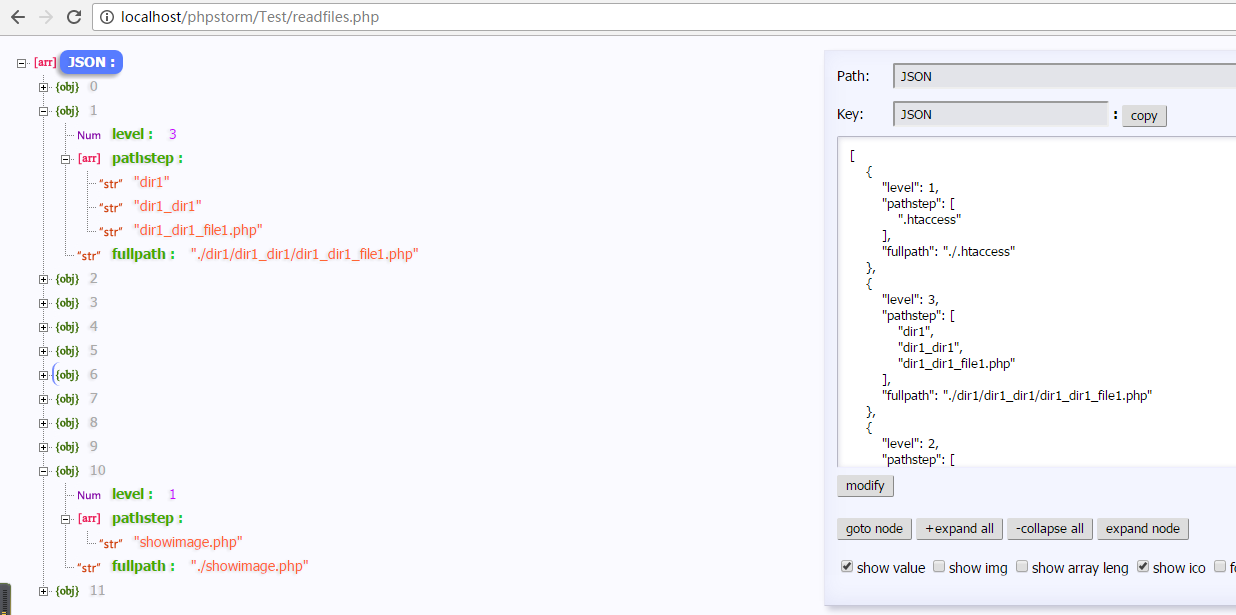### 父级目录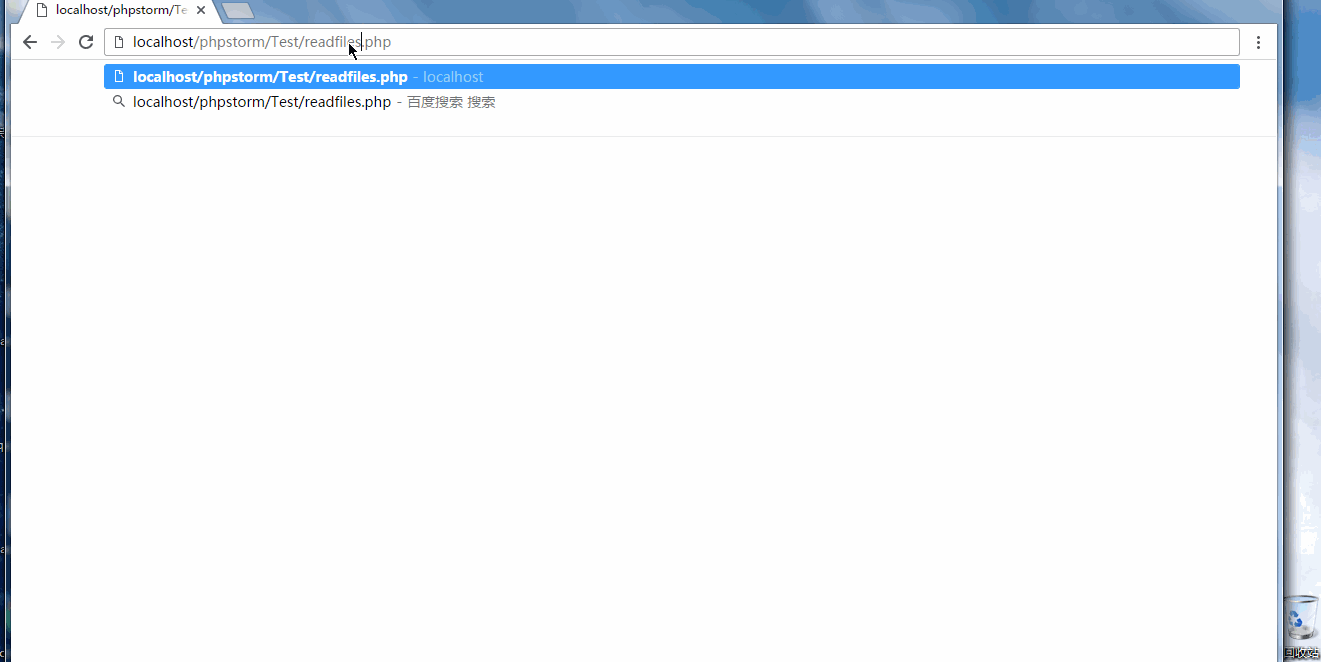## 总结

©️2019 CSDN 皮肤主题: 数字20 设计师: CSDN官方博客